### Submit a Resource

NRICH: At Right Angles

Can you decide whether two lines are perpendicular or not? Can you do this without drawing them?

This problem follows on from How Steep is the Slope?

It’s easy to draw parallel lines – just check that the gradients match.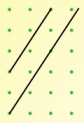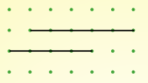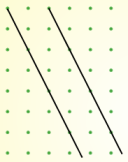Gradient 32 Gradient 0 Gradient −2

But I’m finding it harder to draw perpendicular lines. Here are my best efforts so far but I don’t think they’re quite right!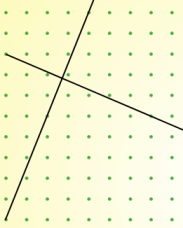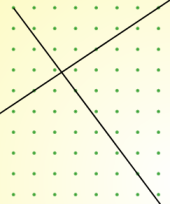I know that the sides of a square are at right angles, so if I learn to draw tilted squares I may be able to find an efficient method for drawing perpendicular lines.

Experiment with the interactivity below until you can draw squares with confidence.
Work out the gradients of the lines which form your squares.
Is there a relationship between the gradients of perpendicular lines?
Can you use your relationship to explain why the two sets of lines above are not perpendicular?

Full Screen Version

Here are some pairs of coordinates which can be joined to make straight lines.
Decide whether the two lines are perpendicular or not, and explain how you know.
Can you decide without plotting the points?
 First line Second line Through (6,9) and (10,1) Through (4,2) and (14,7) Through (6,8) and (21,12) Through (1,4) and (5,14) Through (-3,-2) and (-1,1) Through (6,1) and (15,-5)

Age 14 to 16

Math Topics
Algebra & Pre-Algebra, Functions & Graphs, Linear Equations
High School, Educator

## Organization

NRICH (University of Cambridge)

## Type of Resource

Challenge
Online Interactive Activity